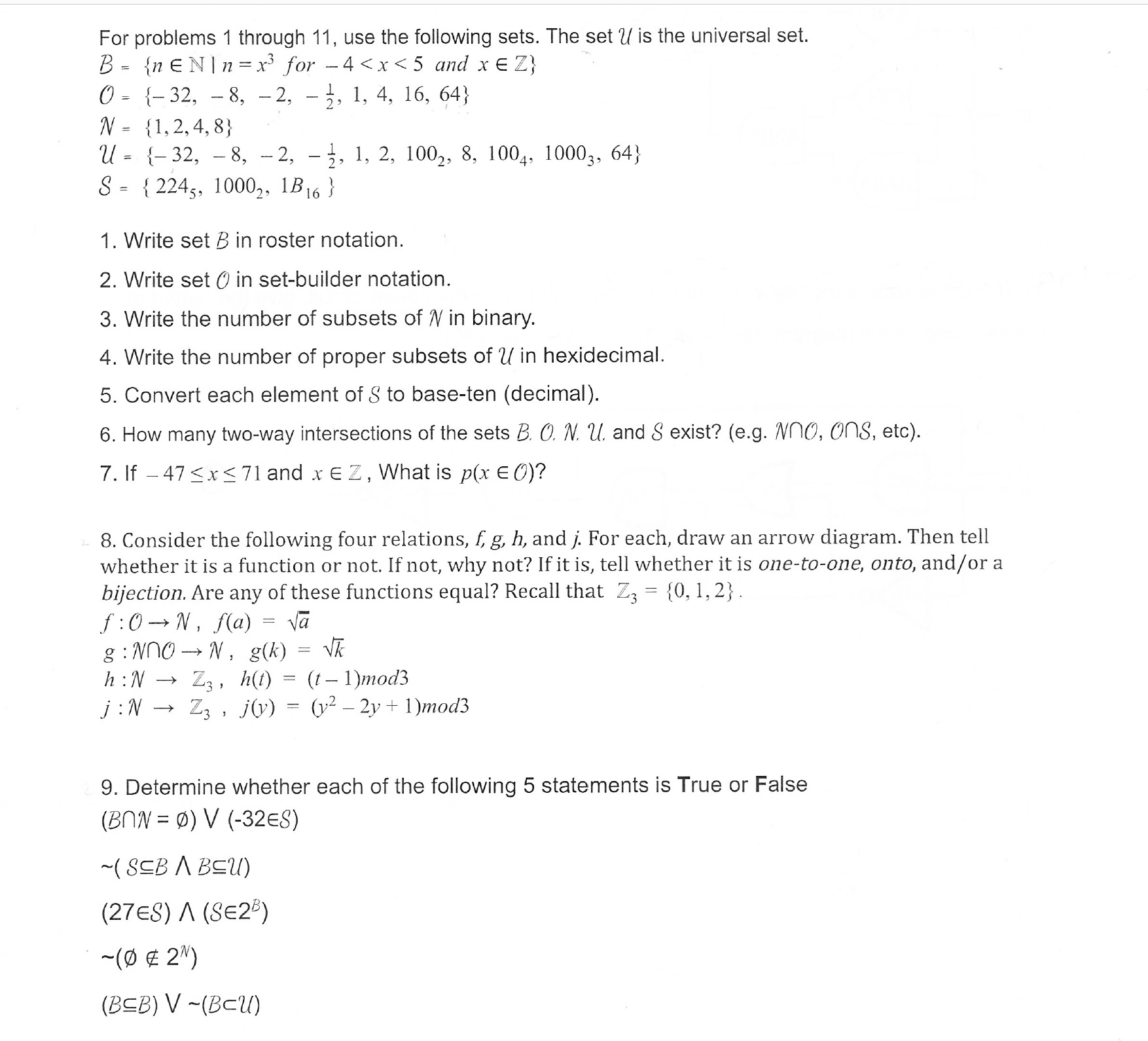For problems 1 through 11, use the following sets. The set U is the universal set.0- -32, - 8, -2, - 3, 1, 4, 16, 64)N-1,2,4,8)U= {-32,-8,-2, .. 1, 2, 100-, 8. 1004. 10003, 64)S-2245, 1000,, B16)1. Write set B in roster notation.2. Write set O in set-builder notation3. Write the number of subsets of N in binary4. Write the number of proper subsets of U in hexidecimal.5. Convert each element of S to base-ten (decimal)6. How many two-way intersections of the sets B. O. N u. and S exist? (e.g. Nno, ons, etc)7. If -47

Question

I only need the explanation of number 8. I do not know how to do it. Thankshelp_outlineImage TranscriptioncloseFor problems 1 through 11, use the following sets. The set U is the universal set. 0- -32, - 8, -2, - 3, 1, 4, 16, 64) N-1,2,4,8) U= {-32,-8,-2, .. 1, 2, 100-, 8. 1004. 10003, 64) S-2245, 1000,, B16) 1. Write set B in roster notation. 2. Write set O in set-builder notation 3. Write the number of subsets of N in binary 4. Write the number of proper subsets of U in hexidecimal. 5. Convert each element of S to base-ten (decimal) 6. How many two-way intersections of the sets B. O. N u. and S exist? (e.g. Nno, ons, etc) 7. If -47
Step 1

As per norms, the subparts for question 8 are answered.To analyze the given relation f.

Step 2

f , being a relation from O to N is a subset of O x N (the cartesian product). As f is defined as square root, the elements -32,-8,-2 and -1/2 are n...

Want to see the full answer?

See Solution

Want to see this answer and more?

Our solutions are written by experts, many with advanced degrees, and available 24/7

See Solution
Tagged in

Math